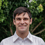This is an archived static version of the original phylobabble.org discussion site.

# [Paper] Efficient Continuous-Time Markov Chain Estimation (in very large state spaces)ematsen

Efficient Continuous-Time Markov Chain Estimation

Monir Hajiaghayi, Bonnie Kirkpatrick, Liangliang Wang, Alexandre Bouchard-Côté http://jmlr.org/proceedings/papers/v32/hajiaghayi14.pdf

Many problems of practical interest rely on Continuous-time Markov chains~(CTMCs) defined over combinatorial state spaces, rendering the computation of transition probabilities, and hence probabilistic inference, difficult or impossible with existing methods. For problems with countably infinite states, where classical methods such as matrix exponentiation are not applicable, the main alternative has been particle Markov chain Monte Carlo methods imputing both the holding times and sequences of visited states. We propose a particle-based Monte Carlo approach where the holding times are marginalized analytically. We demonstrate that in a range of realistic inferential setups, our scheme dramatically reduces the variance of the Monte Carlo approximation and yields more accurate parameter posterior approximations given a fixed computational budget. These experiments are performed on both synthetic and real datasets, drawing from two important examples of CTMCs having combinatorial state spaces: string-valued mutation models in phylogenetics and nucleic acid folding pathways.

The first important thing is to figure out how to calculate the transition probability of an x to a y given that some change occurs in the case when the state space is very big. String-valued processes fall in this category, for example. They bias things with a potential:Second, one needs to marginalize out the event (i.e. jump) times. This is done by constructing a CTMC such that the difficult part of the marginalization are the transition probabilities of the CTMC:Alexandre Bouchard-Côté does fantastic work. H/T @cmccoy.